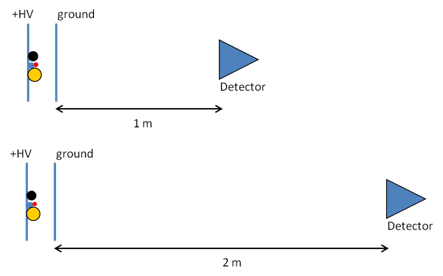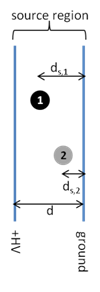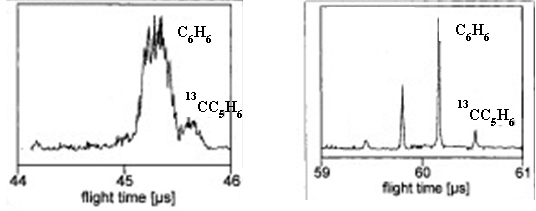# Section 3B. Time of Flight (TOF) Mass Analyzer

$$\newcommand{\vecs}{\overset { \rightharpoonup} {\mathbf{#1}} }$$ $$\newcommand{\vecd}{\overset{-\!-\!\rightharpoonup}{\vphantom{a}\smash {#1}}}$$$$\newcommand{\id}{\mathrm{id}}$$ $$\newcommand{\Span}{\mathrm{span}}$$ $$\newcommand{\kernel}{\mathrm{null}\,}$$ $$\newcommand{\range}{\mathrm{range}\,}$$ $$\newcommand{\RealPart}{\mathrm{Re}}$$ $$\newcommand{\ImaginaryPart}{\mathrm{Im}}$$ $$\newcommand{\Argument}{\mathrm{Arg}}$$ $$\newcommand{\norm}{\| #1 \|}$$ $$\newcommand{\inner}{\langle #1, #2 \rangle}$$ $$\newcommand{\Span}{\mathrm{span}}$$ $$\newcommand{\id}{\mathrm{id}}$$ $$\newcommand{\Span}{\mathrm{span}}$$ $$\newcommand{\kernel}{\mathrm{null}\,}$$ $$\newcommand{\range}{\mathrm{range}\,}$$ $$\newcommand{\RealPart}{\mathrm{Re}}$$ $$\newcommand{\ImaginaryPart}{\mathrm{Im}}$$ $$\newcommand{\Argument}{\mathrm{Arg}}$$ $$\newcommand{\norm}{\| #1 \|}$$ $$\newcommand{\inner}{\langle #1, #2 \rangle}$$ $$\newcommand{\Span}{\mathrm{span}}$$

This is set of questions guides students through the principle of a TOF mass analyzer. There is very little reading and all questions are for small group discussion.

(Source for figures and animations: Dr. Jon Karty of Indiana University Mass Spectrometry Facility)Consider the diagram above, which shows 4 ions (the circles), between two electrical plates. The ions have different masses and higher mass is represented by a larger circle. Imagine the entire schematic is contained in a long tube under vacuum. After the ions enter, the left plate is brought to a positive high voltage (+HV) such as +15,000 V. The right plate is a grid, like a screen door, so that ions can pass through, and is held at electrical ground (0 V).

1. In this scenario, the ions will accelerate once the +HV is applied. If the ions are positively charged, which direction will they move?
2. If all of the ions have a charge of +1, which ion will reach the detector first? The larger circles represent ions with higher mass.
3. What factors other than mass will influence the velocity of the ions?
4. This time of flight mass analyzer uses an applied voltage (+HV) to give kinetic energy (KE) to the ions. The amount of energy imparted to the ions is given by Equation $$\ref{1}$$:

$KE = zV \label{1}$

where z is the charge on the ion and V is the magnitude of the high voltage (HV).

2. If all of the ions have the same charge, what will be true about their kinetic energies?

5. You may remember another equation for kinetic energy from physics:

$KE = \dfrac{1}{2} mv^2 \label{2}$

where m is the mass of the object and v is its velocity.

1. Consider the four ions at the start of this worksheet. Which ion will have the highest velocity?

2. What is the order of ions reaching the detector?

3. Is the TOF mass analyzer dispersive or scanning?

4. Why do you think it is called a “time-of-flight” mass analyzer?

5. Consider a +1 ion with m/z = 115 which enters the time-of-flight source region (between the two plates).

If +HV = 2000 V, how much kinetic energy is imparted to the ion in Joules?

1 elementary charge = 1.6 × 10-19 C $$\mathrm{1\: V = 1\:\: \large{ {}^J/_C} }$$

6. What is the velocity of the ion in m/s?

$$\mathrm{1\: J = 1\: \dfrac{kg \cdot m^2}{s^2}}$$ 1 amu = 1.66 × 10-27 kg

7. If the flight tube is 2 m long, how long will the ion take to reach the detector? Remember that v = d/t, where v is velocity, d is distance traveled, and t is time.
6. The ability of a mass spectrometer to distinguish between two different m/z ions is called resolving power.

A common definition for resolving power is the m/z of the peak (m) divided by the width of the peak at half maximum height (Δm).

$$\textrm{Resolving Power} = \dfrac{m}{\Delta m}$$Image adapted from UC Davis Fiehn Metabolomics Lab. fiehnlab.ucdavis.edu/projects...ass_Resolution

1. Calculate the resolving power of the mass analyzer from the peak in the figure.
2. Using the resolving power calculated in part (a), sketch the appearance of the two peaks with m/z ratios of 1500 and 1500.5. Would you say these peaks are resolved?
7. Consider the two instrument schematics for a time of flight mass analyzer below. Which one do you think will be better at resolving small differences in the masses of the ions? Explain.8. Consider the possibility diagrammed below, in which two ions of the same mass and charge start at slightly different positions in the source region.1. Which ion will leave the source region with the higher velocity, Ion 1 or Ion 2?

Equation $$\ref{1}$$ can also be written as:

$KE=zEd_s \tag{3.1b}$

where E is the electric field (V/d) and ds is the distance traveled in the source region. Use this equation to explain which ion (1 or 2) will leave the source with the highest velocity.

2. How will the position of different ions in the source affect the resolving power of the mass analyzer? Draw a peak for a given m/z similar to the one in question 7 assuming that the position of the ion in the source did not affect kinetic energy. Draw a new peak taking into account how different positions of ions in the source affect the kinetic energy of the ions.

9. Consider the instrument diagram below, which adds a “reflectron” to the mass analyzer. The reflectron consists of a series of grids held at increasingly high positive potentials from ground (0 V) up to +HV2. Note: +HV2 > +HV1.1. On the diagram above, sketch the flight paths for Ion 1 and Ion 2 (these ions have the same m/z ratio and are the same ions in question 8). Note that the reflectron is angled toward the detector.
2. Which ion penetrates farther into the reflectron?
3. How does this arrangement correct for the ions’ different starting positions in the source?
10. Examine the diagram of a reflectron TOF mass analyzer.1. What is the order of ions reaching the detector in the reflectron time of flight mass spectrometer? (all ions have a +1 charge)
2. Why is the ionization method MALDI frequently coupled with a time of flight mass analyzer?
3. Examine the two mass spectra below. Identify which spectrum is from a reflectron TOF instrument and which is from a linear TOF instrument?Section 3B. Time of Flight (TOF) Mass Analyzer is shared under a not declared license and was authored, remixed, and/or curated by LibreTexts.